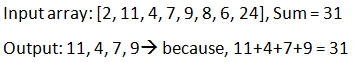# Four elements that sum to given

## In the given array, find the set of four elements whose sum is equal to given value k.

### Example## Algorithm

Time complexity: O(n2logn)

a. Create a struct which stores two elements and sum of the elements.

b. Create an auxiliary array of size (n)(n-1)/2, in the auxiliary array store all possible pairs from the input array and sum of them.

c. So, instead of finding all the four elements. We need to find a pair of elements in the new auxiliary whose sum equal to sum.

d. For finding the pair, sort the array based on the difference between sums.

e. Find the pair now using same algorithm to find the pair of elements to given sum.
1) Maintain two position one at the end of the array and one at start.
2) Move in the array to find the sum, if current sum > sum move last position.
3) Else, move first one

f. Finally print all the elements whose sum equal to given value.

## C++ Program

``````#include <stdio.h>
#include <stdlib.h>

struct pair
{
int first_element;
int second_element;
int Sum;
};
int compareSums(const void *a, const void * b)
{
return ( (*(pair *)a).Sum - (*(pair*)b).Sum );
}

bool isThereCommon(struct pair a, struct pair b)
{
if (a.first_element == b.first_element || a.first_element == b.second_element || a.second_element == b.first_element || a.second_element == b.second_element)
{
return false;
}
return true;
}

void FindFour(int array[], int n, int X)
{
int i, j;
int size = (n*(n-1))/2;
//auxilary array to store sums of elements
struct pair auxiliary_array[size];
int k = 0;
for (i = 0; i < n-1; i++)
{
for (j = i+1; j < n; j++)
{
auxiliary_array[k].Sum = array[i] + array[j];//sum of elements
auxiliary_array[k].first_element = i;//index of first element
auxiliary_array[k].second_element = j;//index of second element
k++;
}
}
qsort(auxiliary_array, size,sizeof(auxiliary_array),compareSums);
i = 0;
j = size-1;
while (i < size && j >=0 )
{
if ((auxiliary_array[i].Sum + auxiliary_array[j].Sum == X) && isThereCommon(auxiliary_array[i], auxiliary_array[j]))
{
printf ("%d, %d, %d, %d\n",array[auxiliary_array[i].first_element],array[auxiliary_array[i].second_element],array[auxiliary_array[j].first_element],array[auxiliary_array[j].second_element]);
return;
}
else if (auxiliary_array[i].Sum + auxiliary_array[j].Sum > X)
{
j--;
}
else
{
i++;
}
}
}

//Main function
int main()
{
int input_array[] = {10, 20, 30, 40, 1, 2};
int size = sizeof(input_array)/sizeof(input_array);
int Sum = 91;
FindFour(input_array,size,Sum);
return 0;
}``````

Scroll to Top